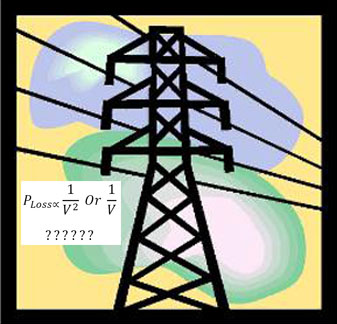In studying of losses in power systems, often spoken that power loss decrease is proportional to the inverse square of system voltage increase. For example, if the voltage is doubled, the losses will be a quarter. It should be noted that this conclusion is correct only when that after increasing the line voltage (and so reducing the line current) conductor cross section remains the same as previous. But this assumption is unreasonable and unwise, because conductor cross section must be chosen based on current amount.